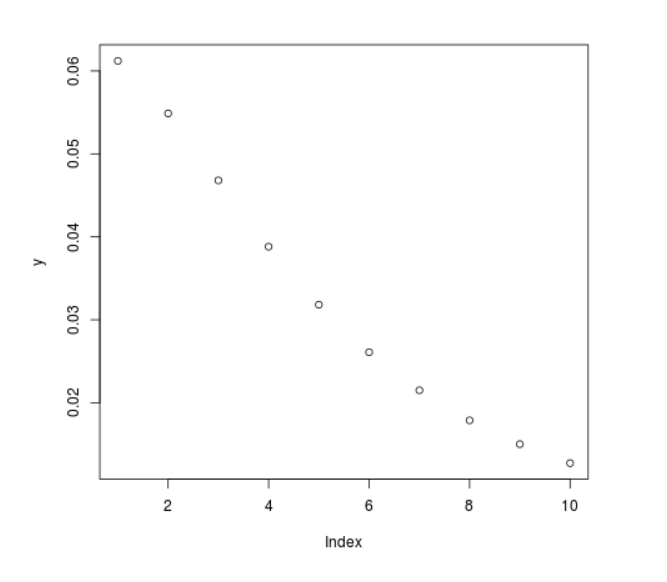# Compute Cauchy Density in R Programming – dcauchy() Function

• Last Updated : 25 Jun, 2020

`dcauchy()` function in R Language is used to calculate the cauchy density. It also creates a density plot of cauchy distribution.

Syntax: dcauchy(vec, scale)

Parameters:
vec: x-values for cauchy function
scale: Scale for plotting

Example 1:

 `# R Program to compute cauchy density`` ` `# Creating vector for x-values``x <``-` `seq(``1``, ``10``, by ``=` `1``)`` ` `# Apply dcauchy() Function``y <``-` `dcauchy(x, scale ``=` `5``)``y`

Output:

```  0.06121344 0.05488101 0.04681028 0.03881828 0.03183099 0.02609097
 0.02150742 0.01788258 0.01501462 0.01273240
```

Example 2:

 `# R Program to compute cauchy density`` ` `# Creating vector for x-values``x <``-` `seq(``1``, ``10``, by ``=` `1``)`` ` `# Apply dcauchy() Function``y <``-` `dcauchy(x, scale ``=` `5``)`` ` `# Plotting a graph``plot(y)`

Output:My Personal Notes arrow_drop_up